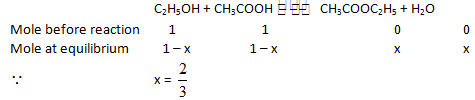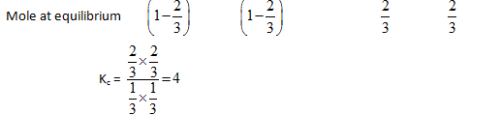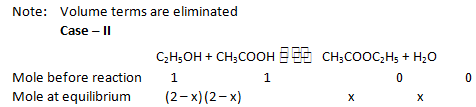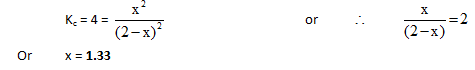JEE  >  Chemical Equation MCQ - 2 (Advanced)

# Chemical Equation MCQ - 2 (Advanced)

Test Description

## 20 Questions MCQ Test Chemistry for JEE Advanced | Chemical Equation MCQ - 2 (Advanced)

Chemical Equation MCQ - 2 (Advanced) for JEE 2022 is part of Chemistry for JEE Advanced preparation. The Chemical Equation MCQ - 2 (Advanced) questions and answers have been prepared according to the JEE exam syllabus.The Chemical Equation MCQ - 2 (Advanced) MCQs are made for JEE 2022 Exam. Find important definitions, questions, notes, meanings, examples, exercises, MCQs and online tests for Chemical Equation MCQ - 2 (Advanced) below.
 1 Crore+ students have signed up on EduRev. Have you?
*Multiple options can be correct
Chemical Equation MCQ - 2 (Advanced) - Question 1

### Solid ammonium carbamate, NH4 CO2NH2 (s) dissociates into ammonia and carbon dioxide when it evaporates as shown by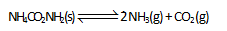'the total pressure of the gases in equilibrium with the solid is 0.116 atm. If 0.1 atm of CO2 is introduced after equilibrium is reached then :

Detailed Solution for Chemical Equation MCQ - 2 (Advanced) - Question 1

the pressure of NHL will decrease due to addition of CO2 (backward, shifting Le-chatelies's principle. The pressure of C02 will be more than 0.1 atm.

*Multiple options can be correct
Chemical Equation MCQ - 2 (Advanced) - Question 2

### For the equilibrium, PCI3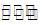PCI3 (g) + CI2 (g) Which of the following sketch may represent above equilibrium. Assume equilibrium can be achieved from either side and by taking any one or more components initially. (Given Kcfor the reaction < 2)

Detailed Solution for Chemical Equation MCQ - 2 (Advanced) - Question 2

Given Kc < 2 therefore in case of B and C Kc = 2*4/6=4/3

and concentration of PCI3 and Cl2 together will decrease or increase as reaction can go in the forward or backward direction

*Multiple options can be correct
Chemical Equation MCQ - 2 (Advanced) - Question 3

### 138 gm of N2O4 (g) is placed in 8.2L container at 300 K. The equilibrium vapour density of mixture was found to be 30.67. Then (R = 0.082 L atm mol-1K–1)

Detailed Solution for Chemical Equation MCQ - 2 (Advanced) - Question 3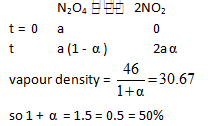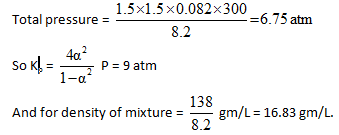*Multiple options can be correct
Chemical Equation MCQ - 2 (Advanced) - Question 4

Solid ammonium carbamate dissociate to give ammonia and carbon dioxide as follows NH2COONH4(s)2NH3(g) + CO2(g)

which of the following graph incorrectly represents the equilibrium.

*Multiple options can be correct
Chemical Equation MCQ - 2 (Advanced) - Question 5

2CaSO4 (s) ˆˆ ˆˆ ˆˆˆˆ 2Ca0(s) + 2SO2(g) + O2(g), ΔH > 0

Above equilibrium is established by taking sufficient amount of CaSO4(s) in a closed container at 1600 K. Then which of the following may be correct option(Assume thatsolid CaSO4 is present in the container in each case).

Detailed Solution for Chemical Equation MCQ - 2 (Advanced) - Question 5

(A) As reaction is endothermic therefore it will go in the forward direction hence moles of Ca0 will increase.

(C) With the increase or decrease of volume particle pressure of the gases will remain same.

(D) Due to the addition of inest gas at constant pressure reaction will proceede in the direct in which move number of gaseous moles are formed.

*Multiple options can be correct
Chemical Equation MCQ - 2 (Advanced) - Question 6

CuSO4 5H2O(s)CuSO4(s) + 5H2O(g)

Kp = 10-10 (atm). 10–2 moles of CuSO4 5H2O(s) is taken in a 2.5L container at 27°C then at equilibrium [Take : R = 1/12 litre atm mol–1 K–1]

Detailed Solution for Chemical Equation MCQ - 2 (Advanced) - Question 6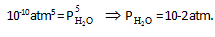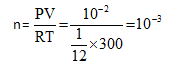*Multiple options can be correct
Chemical Equation MCQ - 2 (Advanced) - Question 7

A closed jar having waters vapours in equilibrium with liquid suddenly all the vapours of the jar is transferred to another,identical jar and is subjected to compression. Assume initial temperature to be the same and negligible volume occupied by the liquid water. Select the observation in the record jar

*Multiple options can be correct
Chemical Equation MCQ - 2 (Advanced) - Question 8

For a reversible reaction ad + bB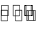cC + dD) ; the variation of K with temperature is

given by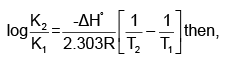*Multiple options can be correct
Chemical Equation MCQ - 2 (Advanced) - Question 9

If two gases ABA and 62C are mixed the following equilibria are readily Established

AB2 (9) + B2C(g) → AB3 (g) + BC (g)

BC(g) + B2C(g) → B3C2 (g)

It the reaction is started only with AB2 with B2C, then which of the following is necessarily true at equilibrium:

Detailed Solution for Chemical Equation MCQ - 2 (Advanced) - Question 9

Let reactions is started with a mole of AB2 and b mole of B2C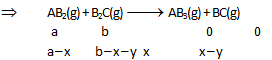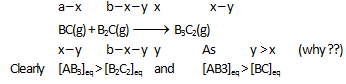*Multiple options can be correct
Chemical Equation MCQ - 2 (Advanced) - Question 10

The following reaction attains equilibrium at high temperature

.N2(g) + 2H2 OP(g) + heat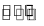2NO(g) + 2H2 (g)

The yield of NO is affected by

Detailed Solution for Chemical Equation MCQ - 2 (Advanced) - Question 10

As concentration of reaction (gaseous) are increased at equilibrium reaction will go in the forward direction. Also above reaction is endothermic therefore increase in temperature will favoaur it.

Chemical Equation MCQ - 2 (Advanced) - Question 11

If a systemat equilibrium is subjected to a change of any one of the factors such as concentration, pressure temperature, the system adjusts itself in such a way as to (Nulify) the effect of that change.
Change of pressure : If a system in equilibrium consists of gases, then the concentrations of all the components can be altered by changing the pressure. When the pressure on the system is increased, the volume decreases proportionately. The total number of moles per unit volume will now be more and the equilibirum will shift in the direction in which there is decrease in number of moles ice., towards the direction in which there is decrease in volume.
Effect of pressure on melting point : There are two types of solids : (a) Solids whose volume decreases on melting, egg., ice, diamond, carborundum, magnesium nitride and quartz Solid (higher volume)Liquid (lower volume) Them process of melting is facilitated at high pressure, thus melting point is lowered. .(b) Solids whose volume increase on melting, e.g., Fe, Cu, Ag, Au, etc.
Solid (lower volume)Liquid (higher volume) In this case the process of melting become difficult at high pressure; thus melting point becomes
high.

(c) Solubility of substances : When solid substance are dissolved in water, either heat is evolved (exothermic) or heat is absorbed (endothermic).
KCI + aqKCl(aq) – heat In such cases, solubility increase with increase in temperature. Consider the case of KOH; when this is dissolved, heat is evolved.
KOH + aqKOH(aq) + heat In such cases, solubility decrease with increase in temperature. (d) Solubility of gases in liquids : When a gas dissolves in liquid, there is decrease in volume. Thus, increase of pressure will favour the dissolution of gas in liquid.
Effect of temperature : Le-Chatelier's principle predicts a system at equilibrium will tend to shift in the endothermic direction when temperature is raised, for then energy is absorbed as heat and the rise in temperature is opposed. Conversely, an equilibrium will shift in the exothermic direction if the temperature is lowered, for then that energy is released and the reduction in temperature is opposed. Van't Hoff equation shows the dependence of equilibrium constant K on temperature as: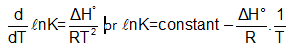Q.

A gas 'X when dissolved in water heat is evolved. Then solubluty of 'X' will                        Increase

Detailed Solution for Chemical Equation MCQ - 2 (Advanced) - Question 11

Solubility of gas is favourable at high pressure and this process is exothermic hence solubility will be more at low temperature.

Chemical Equation MCQ - 2 (Advanced) - Question 12

If a systemat equilibrium is subjected to a change of any one of the factors such as concentration, pressure temperature, the system adjusts itself in such a way as to (Nulify) the effect of that change.
Change of pressure : If a system in equilibrium consists of gases, then the concentrations of all the components can be altered by changing the pressure. When the pressure on the system is increased, the volume decreases proportionately. The total number of moles per unit volume will now be more and the equilibirum will shift in the direction in which there is decrease in number of moles ice., towards the direction in which there is decrease in volume.
Effect of pressure on melting point : There are two types of solids : (a) Solids whose volume decreases on melting, egg., ice, diamond, carborundum, magnesium nitride and quartz Solid (higher volume)Liquid (lower volume) Them process of melting is facilitated at high pressure, thus melting point is lowered. .(b) Solids whose volume increase on melting, e.g., Fe, Cu, Ag, Au, etc.
Solid (lower volume)Liquid (higher volume) In this case the process of melting become difficult at high pressure; thus melting point becomes
high.

(c) Solubility of substances : When solid substance are dissolved in water, either heat is evolved (exothermic) or heat is absorbed (endothermic).
KCI + aqKCl(aq) – heat In such cases, solubility increase with increase in temperature. Consider the case of KOH; when this is dissolved, heat is evolved.
KOH + aqKOH(aq) + heat In such cases, solubility decrease with increase in temperature. (d) Solubility of gases in liquids : When a gas dissolves in liquid, there is decrease in volume. Thus, increase of pressure will favour the dissolution of gas in liquid.
Effect of temperature : Le-Chatelier's principle predicts a system at equilibrium will tend to shift in the endothermic direction when temperature is raised, for then energy is absorbed as heat and the rise in temperature is opposed. Conversely, an equilibrium will shift in the exothermic direction if the temperature is lowered, for then that energy is released and the reduction in temperature is opposed. Van't Hoff equation shows the dependence of equilibrium constant K on temperature as:Q.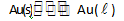Above equilibrium is favoured at :

Detailed Solution for Chemical Equation MCQ - 2 (Advanced) - Question 12

Since density of gold decreases after melting therefore it is favourable at low pressure and high temperature.

*Multiple options can be correct
Chemical Equation MCQ - 2 (Advanced) - Question 13

If a systemat equilibrium is subjected to a change of any one of the factors such as concentration, pressure temperature, the system adjusts itself in such a way as to (Nulify) the effect of that change.
Change of pressure : If a system in equilibrium consists of gases, then the concentrations of all the components can be altered by changing the pressure. When the pressure on the system is increased, the volume decreases proportionately. The total number of moles per unit volume will now be more and the equilibirum will shift in the direction in which there is decrease in number of moles ice., towards the direction in which there is decrease in volume.
Effect of pressure on melting point : There are two types of solids : (a) Solids whose volume decreases on melting, egg., ice, diamond, carborundum, magnesium nitride and quartz Solid (higher volume)Liquid (lower volume) Them process of melting is facilitated at high pressure, thus melting point is lowered. .(b) Solids whose volume increase on melting, e.g., Fe, Cu, Ag, Au, etc.
Solid (lower volume)Liquid (higher volume) In this case the process of melting become difficult at high pressure; thus melting point becomes
high.

(c) Solubility of substances : When solid substance are dissolved in water, either heat is evolved (exothermic) or heat is absorbed (endothermic).
KCI + aqKCl(aq) – heat In such cases, solubility increase with increase in temperature. Consider the case of KOH; when this is dissolved, heat is evolved.
KOH + aqKOH(aq) + heat In such cases, solubility decrease with increase in temperature. (d) Solubility of gases in liquids : When a gas dissolves in liquid, there is decrease in volume. Thus, increase of pressure will favour the dissolution of gas in liquid.
Effect of temperature : Le-Chatelier's principle predicts a system at equilibrium will tend to shift in the endothermic direction when temperature is raised, for then energy is absorbed as heat and the rise in temperature is opposed. Conversely, an equilibrium will shift in the exothermic direction if the temperature is lowered, for then that energy is released and the reduction in temperature is opposed. Van't Hoff equation shows the dependence of equilibrium constant K on temperature as:Q.

For the reaction,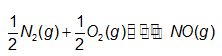pressure is increased by reducing the volume of the container then

Chemical Equation MCQ - 2 (Advanced) - Question 14

If a systemat equilibrium is subjected to a change of any one of the factors such as concentration, pressure temperature, the system adjusts itself in such a way as to (Nulify) the effect of that change.
Change of pressure : If a system in equilibrium consists of gases, then the concentrations of all the components can be altered by changing the pressure. When the pressure on the system is increased, the volume decreases proportionately. The total number of moles per unit volume will now be more and the equilibirum will shift in the direction in which there is decrease in number of moles ice., towards the direction in which there is decrease in volume.
Effect of pressure on melting point : There are two types of solids : (a) Solids whose volume decreases on melting, egg., ice, diamond, carborundum, magnesium nitride and quartz Solid (higher volume)Liquid (lower volume) Them process of melting is facilitated at high pressure, thus melting point is lowered. .(b) Solids whose volume increase on melting, e.g., Fe, Cu, Ag, Au, etc.
Solid (lower volume)Liquid (higher volume) In this case the process of melting become difficult at high pressure; thus melting point becomes
high.

(c) Solubility of substances : When solid substance are dissolved in water, either heat is evolved (exothermic) or heat is absorbed (endothermic).
KCI + aqKCl(aq) – heat In such cases, solubility increase with increase in temperature. Consider the case of KOH; when this is dissolved, heat is evolved.
KOH + aqKOH(aq) + heat In such cases, solubility decrease with increase in temperature. (d) Solubility of gases in liquids : When a gas dissolves in liquid, there is decrease in volume. Thus, increase of pressure will favour the dissolution of gas in liquid.
Effect of temperature : Le-Chatelier's principle predicts a system at equilibrium will tend to shift in the endothermic direction when temperature is raised, for then energy is absorbed as heat and the rise in temperature is opposed. Conversely, an equilibrium will shift in the exothermic direction if the temperature is lowered, for then that energy is released and the reduction in temperature is opposed. Van't Hoff equation shows the dependence of equilibrium constant K on temperature as:Q.

The plot of log K against 1 -- is a straight line with positive slope (K being the equilibrium constant of a reaction), which of the following is then correct ?

Detailed Solution for Chemical Equation MCQ - 2 (Advanced) - Question 14

Since the slope of the straight line graph between log and 1/2
is positive, ΔHreaction would be negative, i.e.,
the forward reaction would be exothermic.

Chemical Equation MCQ - 2 (Advanced) - Question 15

In gaseous dissociation reactions, the total mass remains unchanged and the number of moles increases as a result of the reaction. Thus the average molecular weight and hence the vapour density decreases. In other words, the volume increases at constant temperature and pressure and hence the vapour density decreases.The relationship between vapour density in the beginning and vapour density at equilibrium can be found as follows:

At constant temperature and constant pressure for a fixed mass of gaseous mixtures,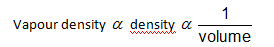PV = nRT Volume ∝ number of moles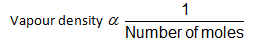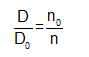Where Do and D are the vapour densities in the beginning and at equilibrium and no and n are the number of moles in the beginning and at equilibrium.The vapour density of PCI5 at 200°C and 252°C are 70.2 and 57.2 respectively at one atmosphere

Q.

The observation shows that the dissociation constants (KP) for the reaction are

Detailed Solution for Chemical Equation MCQ - 2 (Advanced) - Question 15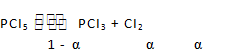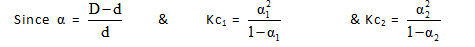Hence Kp for different conditions can be calculated and kp = 0.307 and 1.19 respectively at 200°C and 250°C.

Chemical Equation MCQ - 2 (Advanced) - Question 16

In gaseous dissociation reactions, the total mass remains unchanged and the number of moles increases as a result of the reaction. Thus the average molecular weight and hence the vapour density decreases. In other words, the volume increases at constant temperature and pressure and hence the vapour density decreases.The relationship between vapour density in the beginning and vapour density at equilibrium can be found as follows:

At constant temperature and constant pressure for a fixed mass of gaseous mixtures,PV = nRT Volume ∝ number of molesWhere Do and D are the vapour densities in the beginning and at equilibrium and no and n are the number of moles in the beginning and at equilibrium.The vapour density of PCI5 at 200°C and 252°C are 70.2 and 57.2 respectively at one atmosphere

Q.

This observation shows that

Detailed Solution for Chemical Equation MCQ - 2 (Advanced) - Question 16

As Kp value increases with increase in temperature, this implies the reaction is endothermic.

Chemical Equation MCQ - 2 (Advanced) - Question 17

In gaseous dissociation reactions, the total mass remains unchanged and the number of moles increases as a result of the reaction. Thus the average molecular weight and hence the vapour density decreases. In other words, the volume increases at constant temperature and pressure and hence the vapour density decreases.The relationship between vapour density in the beginning and vapour density at equilibrium can be found as follows:

At constant temperature and constant pressure for a fixed mass of gaseous mixtures,PV = nRT Volume ∝ number of molesWhere Do and D are the vapour densities in the beginning and at equilibrium and no and n are the number of moles in the beginning and at equilibrium.The vapour density of PCI5 at 200°C and 252°C are 70.2 and 57.2 respectively at one atmosphere

Q.

From the given data, it can be interpreted that .

Detailed Solution for Chemical Equation MCQ - 2 (Advanced) - Question 17

More the degree of dissociation, less is the vapour density of PCI5.

Chemical Equation MCQ - 2 (Advanced) - Question 18

The rate of chemical reaction at a particular temperature is proportional to the product of the molar concentration of reactants with each concentration term raised to the power equal to the number of molecules of the respective reactant taking part in the reaction. aA + bB → products, rate of reaction a [Aka [Bib = k [Aka [Bib, where k is the rate constant of the reaction.
EQUILIBRIUM CONSTANT (k) For a general reaction aA + bB <=> cC + dD, forward rate rf = kf [A]a [B]b, backward rate rb = kb [Cicpyi , concentrations of reactants & products at equilibrium are related by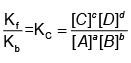where all the concentrations are expressed in mole/liter.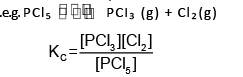In the expression of equilibrium constant those components are kept whose concentration changes with time.
If equilibrium is estabilished by taking all the components in the reaction then to predict the direction of reactions we calculate the reaction quotient (Q).

The values of expression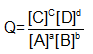at any time during reaction is called reaction quotient if Q > Kc reaction proceed in backward direction until equilibrium in reached if Q < Kc reaction will proceed in forward direction until equilibrium is established if Q = KC Reaction is at equilibrium

Q.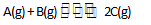Initial concentration of 'A’ is twice the initial concentration of `B'. At equilibrium  concentration of 'A and 'a are same then equilibrium constant for the reaction is

Detailed Solution for Chemical Equation MCQ - 2 (Advanced) - Question 18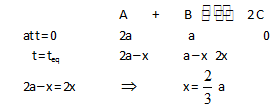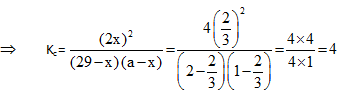Chemical Equation MCQ - 2 (Advanced) - Question 19

The rate of chemical reaction at a particular temperature is proportional to the product of the molar concentration of reactants with each concentration term raised to the power equal to the number of molecules of the respective reactant taking part in the reaction. aA + bB → products, rate of reaction a [Aka [Bib = k [Aka [Bib, where k is the rate constant of the reaction.
EQUILIBRIUM CONSTANT (k) For a general reaction aA + bB <=> cC + dD, forward rate rf = kf [A]a [B]b, backward rate rb = kb [Cicpyi , concentrations of reactants & products at equilibrium are related bywhere all the concentrations are expressed in mole/liter.In the expression of equilibrium constant those components are kept whose concentration changes with time.
If equilibrium is estabilished by taking all the components in the reaction then to predict the direction of reactions we calculate the reaction quotient (Q).

The values of expressionat any time during reaction is called reaction quotient if Q > Kc reaction proceed in backward direction until equilibrium in reached if Q < Kc reaction will proceed in forward direction until equilibrium is established if Q = KC Reaction is at equilibrium

q.

Above homogeneous reaction is carried out in a 2 litre container at a particular temperature by taking 1 mole each of A, B, C and D respectively. If Kc for the reaction is then equilibrium concentration of C is

Detailed Solution for Chemical Equation MCQ - 2 (Advanced) - Question 19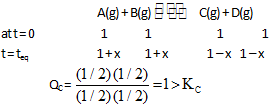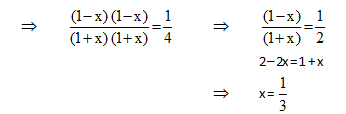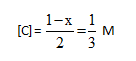Chemical Equation MCQ - 2 (Advanced) - Question 20

The rate of chemical reaction at a particular temperature is proportional to the product of the molar concentration of reactants with each concentration term raised to the power equal to the number of molecules of the respective reactant taking part in the reaction. aA + bB → products, rate of reaction a [Aka [Bib = k [Aka [Bib, where k is the rate constant of the reaction.
EQUILIBRIUM CONSTANT (k) For a general reaction aA + bB <=> cC + dD, forward rate rf = kf [A]a [B]b, backward rate rb = kb [Cicpyi , concentrations of reactants & products at equilibrium are related bywhere all the concentrations are expressed in mole/liter.In the expression of equilibrium constant those components are kept whose concentration changes with time.
If equilibrium is estabilished by taking all the components in the reaction then to predict the direction of reactions we calculate the reaction quotient (Q).

The values of expressionat any time during reaction is called reaction quotient if Q > Kc reaction proceed in backward direction until equilibrium in reached if Q < Kc reaction will proceed in forward direction until equilibrium is established if Q = KC Reaction is at equilibrium

Q.

When C,H5OH and CH3COOH are mixed in equivalent proportion equilibrium is reached when 2/3 of acid and alcohol are used. How much ester will be present when 2 moles of acid were to react with 2 moles of alcohol? .

Detailed Solution for Chemical Equation MCQ - 2 (Advanced) - Question 20